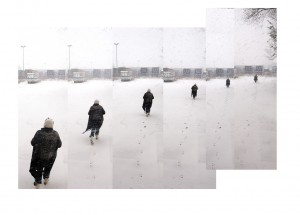# Points: bridges between movementsDid a new version of  ‘a line made by walking’ yesterday. Went through the snow to Tractie and registered myself walking a line in the snow – very little  visual noise because of the whiteness of it all. Documenting this line brought home to me that a line is  a collection of points, really.

So, some thoughts about lines and points:

A point is  the basic entity of geometry. We   think of a point as a “dot” on a piece of paper.  When we draw a point on paper we materialise an immaterial thing. How thick or thin pencil that we use is determines the dimensions, the materialisation of  the point or line. A point is actually a location, rather than a thing.  It might be the point 7 cm from the left edge of a ruler (a point in a one-dimensional space), or it might be the point on your paper that is 15 cm from the top and 19  cm  from the left (a point in a two-dimensional space) , or it might be a point in the middle of the room, 25 cm  off the floor, 120  cm from the left wall, and 160 cm from the front wall (a three-dimensional space).  A point that is moving (me in the pic on the left) is actually a point in a four-dimensional space (the three dimensions plus time). A point itself is a zero-dimensional space – a very God-like thing, in a way. From any point an infinite number of lines may start, an infinite number of walks, of journeys.

Even if there is only one point, one mark on a blank page there is something built into the brain that wills meaning for it. that seeks some kind of relationship or order. If only to use it as a point of orientation in relation to the outline of the page. When  there are two points, immediately the eye will make a connection and “see” a line. When  there are three points, it is unavoidable to interpret them as a triangle; the mind supplies the connections.

A point itself has no size and no thickness.  A point can be the beginning of a line, the end of a line, or the intersection of two lines. A continous surface can be described as a finite collection of lines (this is Deleuze’s striated space), this would be like me describing the surface of Tractie by walking on it. Which I have done, see blog ‘walking a map of Tractie‘ . As a line can be described as a a finite collection of points (that is what I am doing in this photograph), and a finite collection of lines make up a surface, any surface can be described as a finite collection of points (this is a Riemann’s surface).

Points are essential in surveying. Point positioning is the determination of the coordinates of a point on land, at sea, or in space with respect to a coordinate system. From a point with known coordinates, the surveyor works to establish new points by measuring angles and distances to these points. This is called triangulation, as the basic arithmatics are done using the properties of the triangle: three intersecting lines establishing three corner points.

Kandinsky talked about the geometric points in ‘point and line to plane’ and describes it  in terms of sound and silence:  “The geometric point is an invisible thing. Therefore, it must be defined as an incorporeal thing. Considered in terms of substance, it equals zero.  Hidden in this zero, however, are various attributes which are ‘human’ in nature. We think of this zero – the geometric point – in relation to the greatest possible brevity, i.e. to the highest degree of restraint which, nevertheless, speaks. Thus we look upon the geometric point as the ultimate and most singular union of silence and speech”.

By analogy, I think of points in terms of movement and stand-still:  considered in terms of substance, it equals zero. Hidden in this zero, however, are various attributes which are ‘human’ in nature. I  think of this zero – the geometric point – in relati0n to the greatest possible brevity, i.e. to the highest degree of restraint which, nevertheless, moves. Thus we look upon the geometric point as the ultimate and most singular union of stillness and movement’.

The point as the moment (just) before movement starts, or (just) after it ends. The point as the interval between one movement and another, the bridge between movements.